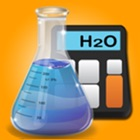iChem Calc - Chemistry Calculators

• SEARCH TYPE

All iPhone applications categories

All iPhone games categoriesiChem Calc - Chemistry Calculators

by: 0 0

Screenshots

Description

Have you got a Chemistry test coming up? Are you preparing a Chemistry assignment or dealing with calculations based on equations and want to make sure your answers are correct before submission? Do you wish you had an easy access to data while performing experiments in the Chemistry laboratory? If yes, then iChemCalc is the application for you. It contains a lot of calculators that can assist students of all grades studying Chemistry.

The application presents a set of convenient and easy to use features that separates it from any other Chemistry calculator application.

CALCULATORS:

The app contains over 60 calculators based on the following six broad categories:

- Physical Chemistry
Moles
Avogardo's Number
Molarity
Molality
Mole fraction
Molecular formula calculations
Percentage yield
Specific Retardation Factor
centigrade to Kelvin scale
Centigrade to Fahrenheit scale
Fahrenheit to Centigrade scale
Co-efficient of viscosity
General gas constant
Density
Density calculations from general gas equation
Partial pressure
Total pressure
Pressure calculations from molecular theory of gas
Root mean square velocity
Average velocity
Most probable velocity
Kinetic energy calculation
Law of mass action
Relationship between Kp and Kc
Rate of reaction
Raoults Law ( equation 1)
Raoults Law ( equation 2)
Raoults Law ( equation 3)
Lowering of vapour pressure
Elevation of boiling point(equation 1)
Elevation of boiling point( equation 2)
Depression in freezing point( equation 1)
Depression in freezing point( equation 2)

- Quantum Mechanics
Planck's equation
Einstein's mass energy relationship
Wave number
Angular momentum
Energy of photon
Radius of orbit simper equation
De-broglie equation
Compton's effect

- Thermodynamics
Pressure volume work
First law of thermodynamics
Enthaply calculations
Measurement of Enthalpy of a reaction( glass calorimeter)
Measurement of Enthalpy of a reaction( bomb calorimeter)

- Inorganic Chemistry
pH calculation
pH calculation from pOH
pOH calculation
pOH calculations from pH
Henderson-Hasselbalch equation For acids
Henderson-Hasselbalch equation For base
Buffer capacity
Bond order
Dipole moment
Percentage of ionic character

- Nuclear Chemistry
Decay constant
Half life
Half life calculations from decay constant

Details of the calculator inputs and outputs are available at all the time for reference.
The formula and image (if applicable) is shown in each calculator, hence making the calculations transparent.

SEARCH:

The app makes use of a unique Search feature so that you don’t have to find your desired calculator going in and out of the categories. You can search a calculator using its name as keywords. The application will show up matching results so that you can swiftly complete the task at hand.

FAVORITES:

The app also lets you bookmark most commonly used calculators as favorites so that you can access your favorite calculators quickly at any time.

If you have any suggestions to improve the application or you wish a specific calculator to be added please drop an email to support@softack.com. New calculators will be added with each update.